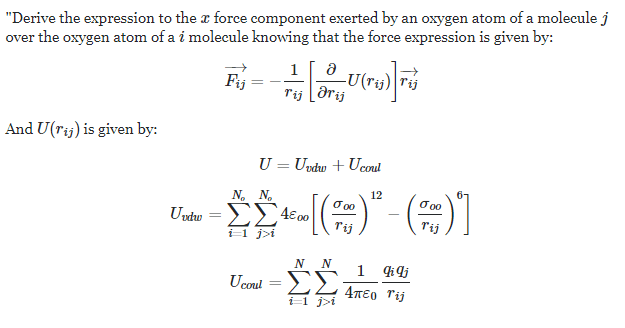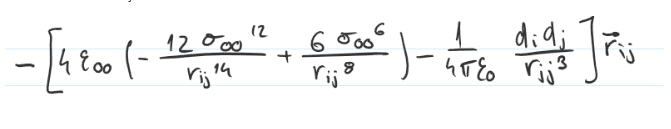# Derivative of a vector

Jorzef
Homework Statement:
Derive the expression to the x force component exerted by an oxygen atom of a molecule j over the oxygen atom of a i molecule knowing that the force expression is given by (equation on attempt at a solution)
Relevant Equations:
Equations on attempt at a solutionHello everyone, I'm stuck doing this problem, I've tackled the partial derivative but i can't figure out the derive for x component part, i solved the partial derivative part, i came to this result:What do can i do from here on, thank you!

Homework Helper
Gold Member
2022 Award
Homework Statement:: Derive the expression to the x force component exerted by an oxygen atom of a molecule j over the oxygen atom of a i molecule knowing that the force expression is given by (equation on attempt at a solution)
Relevant Equations:: Equations on attempt at a solution

View attachment 262781

Hello everyone, I'm stuck doing this problem, I've tackled the partial derivative but i can't figure out the derive for x component part, i solved the partial derivative part, i came to this result:
View attachment 262782
What do can i do from here on, thank you!
"Derive" has two meanings in mathematics. It can mean the differential calculus operation of taking a derivative, or it can simply mean to obtain one equation from others by algebraic means.
I have the feeling you have applied the wrong one.